TitleCollege Algebra
Answer/Discussion to Practice Problems
Tutorial 26: Equations of LinesAnswer/Discussion to 1a Slope = ½ and passes through the origin.

 What are the two things we need to write an equation of a line???? If you said any point on the line and the slope, you are correct.   Do you know what the ordered pair for the origin is?  If you said (0, 0) you are right on!!!  That is the point that we will be using to plug into our equation. Looks like we have all the information we need.  We are ready to put our equation together.  Point/Slope Form:*Point/slope form of the line

 Next, we want to write it in the Slope/Intercept Form, which basically means we need to solve for y: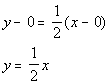*Dist. the 1/2 through (  ) *Slope/intercept form of the line

 The equation of the line that passes through the origin and has a slope of 1/2 is  y - 0 = 1/2(x - 0)   OR   y = 1/2x.Answer/Discussion to 1b Passing through (-2, 4) and (-1, -1).

 What are the two things we need to write an equation of a line???? If you said any point on the line and the slope, you are correct.   We have more than enough points. However, what about the slope?  Does this mean we can't work out the problem?  You are not going to get off that easily.  We do have a way of finding the slope. Tutorial 25: The Slope of a Line shows us how we can get the slope given two points. Let's find that slope: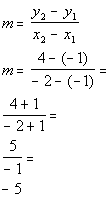*Slope formula *Plug in values  *Simplify

 OK, now we have our slope, which is -5.  Now we want to put the slope and one point into the point/slope equation.  Note that you can use either point to plug in as long as it is a point that the line passes through.  I chose to plug in the point (-2, 4). Point/Slope Form: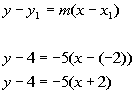*Point/slope form of the line

 Make sure that you are careful when one of your values is negative and you have to subtract it as we did in line 2.  x - (-2)  is not the same as x - 2.

 Next, we want to write it in the Slope/Intercept Form, which basically means we need to solve for y: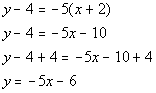*Dist. the -5 through (  ) *Inverse of sub. 4 is add 4 *Slope/intercept form of the line

 The equation of the line that passes through the points (-2, 4) and (-1, -1) is  y - 4 = -5(x + 2)   OR   y = -5x - 6.Answer/Discussion to 1c x-intercept = -5 and y-intercept = -2.

 What are the two things we need to write an equation of a line???? If you said any point on the line and the slope, you are correct. Do you know what the ordered pair is going to be for the x-intercept?  What about the y-intercept?  Above, we leaned that an x-intercept is where the line crosses the x-axis.  That means y's value is always 0.  So the ordered pair for our x-intercept is (-5. 0). Above, we learned that an y-intercept is where the line crosses the y-axis.  That means x's value is always 0.  So the ordered pair for our y-intercept is (0, -2).   We have more than enough points. However, what about the slope?  Does this mean we can't work out the problem?  You are not going to get off that easily.  We do have a way of finding the slope. Tutorial 25: The Slope of a Line shows us how we can get the slope given two points. Let's find that slope: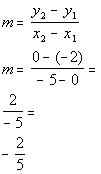*Slope formula *Plug in values  *Simplify

 OK, now we have our slope, which is -2/5.  We want to put the slope and one point into the point/slope equation.  Note that you can use either point to plug in as long as it is a point that the line passes through.  I chose to plug in the point (-5, 0). Point/Slope Form:*Point/slope form of the line

 Make sure that you are careful when one of your values is negative and you have to subtract it as we did in line 2.  x - (-5)  is not the same as x - 5.

 Next, we want to write it in the Slope/Intercept Form, which basically means we need to solve for y:*Dist. the -2/5 through (  ) *Slope/intercept form of the line

 The equation of the line that has an x-intercept of -5 and y-intercept of -2 is y - 0 = -2/5(x + 5)   OR   y = -2/5x - 2.

Last revised on Feb. 6, 2010 by Kim Seward.
All contents copyright (C) 2002 - 2010, WTAMU and Kim Seward. All rights reserved.